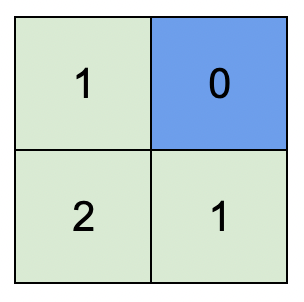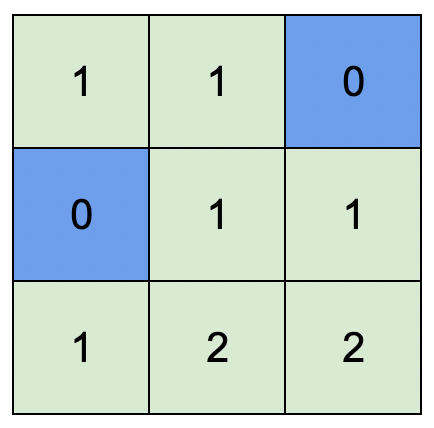Formatted question description: https://leetcode.ca/all/1765.html

# 1765. Map of Highest Peak

Medium

## Description

You are given an integer matrix isWater of size m x n that represents a map of land and water cells.

• If isWater[i][j] == 0, cell (i, j) is a land cell.
• If isWater[i][j] == 1, cell (i, j) is a water cell.

You must assign each cell a height in a way that follows these rules:

• The height of each cell must be non-negative.
• If the cell is a water cell, its height must be 0.
• Any two adjacent cells must have an absolute height difference of at most 1. A cell is adjacent to another cell if the former is directly north, east, south, or west of the latter (i.e., their sides are touching).

Find an assignment of heights such that the maximum height in the matrix is maximized.

Return an integer matrix height of size m x n where height[i][j] is cell (i, j)’s height. If there are multiple solutions, return any of them.

Example 1:Input: isWater = [[0,1],[0,0]]

Output: [[1,0],[2,1]]

Explanation: The image shows the assigned heights of each cell. The blue cell is the water cell, and the green cells are the land cells.

Example 2:Input: isWater = [[0,0,1],[1,0,0],[0,0,0]]

Output: [[1,1,0],[0,1,1],[1,2,2]]

Explanation: A height of 2 is the maximum possible height of any assignment. Any height assignment that has a maximum height of 2 while still meeting the rules will also be accepted.

Constraints:

• m == isWater.length
• n == isWater[i].length
• 1 <= m, n <= 1000
• isWater[i][j] is 0 or 1.
• There is at least one water cell.

## Solution

Do breadth first search starting from all water cells. All water cells have heights 0. For a cell that has height x, if an adjacent cell has not been visited, then the adjacent cell has height x + 1. Since breadth first search finds the shortest distances of the cells from the water cells, it is guaranteed that any two adjacent cells have an absolute height difference of at most 1.

class Solution {
public int[][] highestPeak(int[][] isWater) {
int rows = isWater.length, columns = isWater.length;
int[][] heights = new int[rows][columns];
Queue<int[]> queue = new LinkedList<int[]>();
for (int i = 0; i < rows; i++) {
for (int j = 0; j < columns; j++) {
if (isWater[i][j] == 1) {
heights[i][j] = 0;
queue.offer(new int[]{i, j});
} else
heights[i][j] = -1;
}
}
int[][] directions = { {-1, 0}, {1, 0}, {0, -1}, {0, 1} };
while (!queue.isEmpty()) {
int[] cell = queue.poll();
int row = cell, column = cell;
int height = heights[row][column];
for (int[] direction : directions) {
int newRow = row + direction, newColumn = column + direction;
if (newRow >= 0 && newRow < rows && newColumn >= 0 && newColumn < columns && heights[newRow][newColumn] == -1) {
heights[newRow][newColumn] = height + 1;
queue.offer(new int[]{newRow, newColumn});
}
}
}
return heights;
}
}